2018-06-18 21:42:30 qq_41174320 阅读数 5485
• ###### MATLAB图像处理

全面系统的学习MATLAB在图像处理中的应用

19908 人正在学习 去看看 魏伟

# 一、图像处理预备知识

## 2、图像处理常用方法

### 6）图像识别

Matlab是一个高级的矩阵/阵列语言，它包含控制语句、函数、数据结构、输入和输出和面向对象编程特点。用户可以在命令窗口中将输入语句与执行命令同步，也可以先编写好一个较大的复杂的应用程序（M文件）后再一起运行。

# 三、MATLAB图像处理代码

## 1、图像读取及保存

MATLAB的基本数据单位是矩阵，一幅图像可定义为一个二维函数f(x,y)，其中x和y是空间（平面）坐标，而在任何一对空间坐标(x,y)处的幅值f称为图像在该点处的强度或灰度。当x，y和灰度值f是有限的离散数值时，我们称该图像为数字图像。在MATLAB中，数字图像就是一个个矩阵。

## 1）图像读取

### 2）图像保存

imwrite(OutImg,'d:\photo\yn_ln.jpg'); %将OutImg矩阵表示的图像存储到电脑D盘photo文件夹中，并命名为yn_ln的.jpg格式的图像。

### 3）图像显示

subplot(行数，列数，区域索引);

## subplot(1,1,1);imshow(InImg); %将InImg图像文件显示到屏幕上指定位置

### 4）图像转换

I=rgb2gray(rgbImg)将彩色图像转换为灰度图像，rgbImg为彩色图像
bw=im2bw(Img,level)： 将灰度图像转换为二值图像，Img为灰度图像，level为0~1的灰度级阈值；## 1）数字图像灰度增强

J=imadjust(I,[low high], [bottom top],gamma): 对灰度图像进行直接灰度变换；I为增强前灰度图像，J为增强后的灰度图像，[low high]增强前灰度级范围，[bottom top]增强后灰度级范围

clear;
I=rgb2gray(InImg);
bw1=im2bw(I,0.8);
bw2=im2bw(J,0.8);
bw2=medfilt2(bw2);
subplot(1,2,1);imshow(bw1);
subplot(1,2,2);imshow(bw2);## 2）数字图像空域滤波

S=medfilt2(J): 对图像进行中值滤波

M=0;V=0.01;
J1=imnoise(I, 'gaussian',M,V);
S=medfilt2(J1);
subplot(1, 2, 1); imshow(J1);
subplot(1, 2, 2); imshow(S);## 3）数字图像频域滤波

F=fft2(I): 2D 傅里叶正变换

Pha=angle(F): 计算相位谱

R= abs(F): 计算功率谱
F1=real(F): 获得傅里叶变换实部分量
F2=imag(F): 获得傅里叶变换虚部分量
fftshift(F): 移动傅里叶频谱中心至零频率

I=ifft2(F): 2D 傅里叶逆变换

I=BWBand(21,31);
F=fft2(I);                        %傅里叶正变换
F=fftshift(F);
L=abs(F);
L= mat2gray(L);
I3=imrotate(BWBand(21, 31),30);
F3=fft2(I3);
F3=fftshift(F3);
L3=abs(F3);
L3= mat2gray(L3);
subplot(141); imshow(I);
title('原图');
subplot(142); imshow(I3);
title('旋转30度图');
subplot(143); imshow(L);
title('功率谱图');
subplot(144); imshow(L3);

title('旋转30度功率谱图');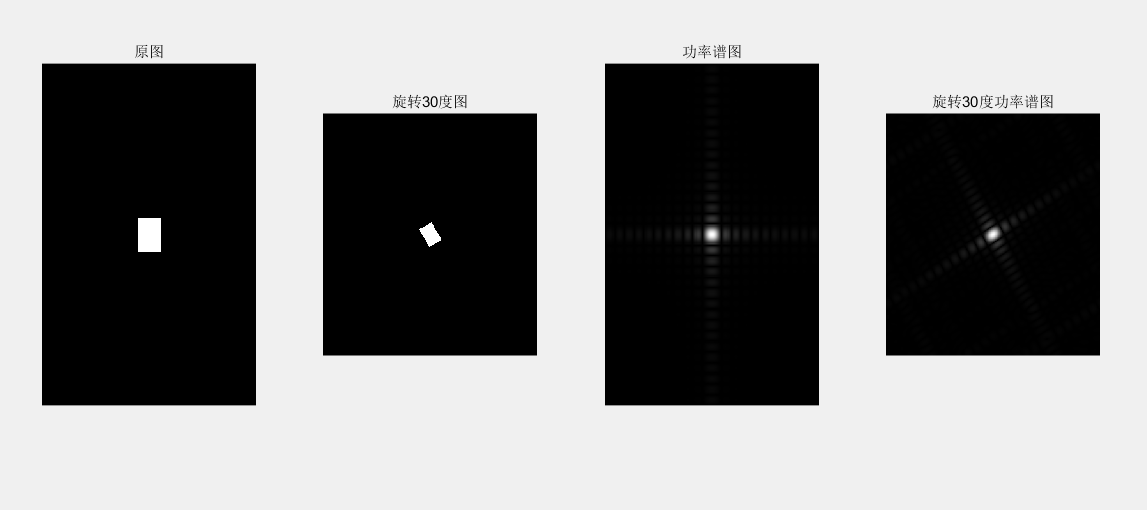## （2）InImg=imread('d:\photo\ying.jpg');subplot(2, 2, 1); imshow(InImg); title('原彩色图');I=rgb2gray(InImg);J1=imnoise(I, 'salt & pepper',0.01);subplot(2, 2, 2); imshow(J1); title('椒盐噪声污染图');[f1, f2]=freqspace([199 200],'meshgrid');        %产生等间隔采样的频率响应R=sqrt(f1.^2+ f2.^2);                   %计算功率谱Hd=ones([199 200]);Hd(R>0.5)=0;                                   %产生理想的带通滤波器，频率带宽[0.1 0.5]win= fspecial('gaussian',[199 200],2); %采用高斯函数生成同样大小的窗函数win= win./max(win(:));                       %窗口函数归一化H=fwind2(Hd,win);                           %利用窗口函数产生实际的带通滤波器 subplot(2, 2, 3); freqz2(H);ff= imfilter (J1,H);subplot(2, 2, 4); imshow(ff);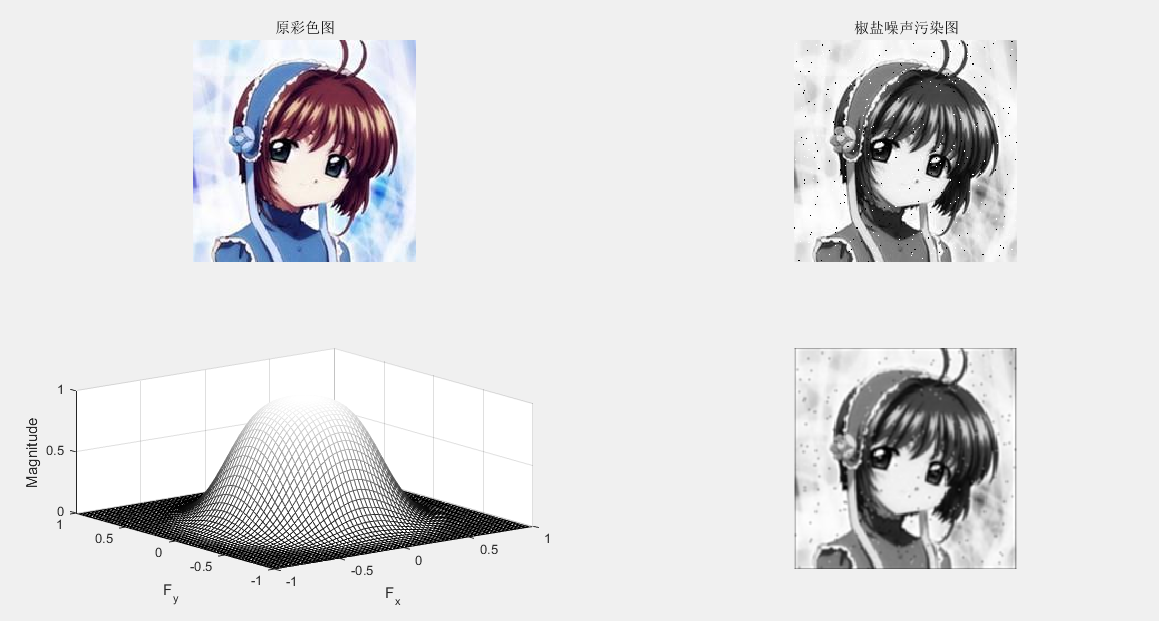## 3、图像复原

J = WIENER2(I,[M N]): 对灰度图像实施维纳自适应滤波 ，[M N]像素领域大小
C=conv2(A,B) : 计算矩阵 A B 的卷积

I=rgb2gray(InImg);
J1=imnoise(I, 'gaussian');
B1=[0 0.2 0;0.2 0.2 0.2; 0 0.2 0];   %平滑滤波器
H = fspecial('average',[5 5]);
Blurred1 = imfilter (J1,H);
H = fspecial('gaussian',[5 5]);
Blurred2 = imfilter (J1,H);
K = wiener2(J1,[5 5]);
subplot(1, 5, 1); imshow(J1) ; %显示噪声图像
title('高斯噪声图像');
subplot(1, 5, 2); imshow(Blurred1) ; %显示均值滤波图像
title('均值滤波图像');
subplot(1, 5, 3); imshow(Blurred2) ; % 显示高斯滤波图像
title('高斯滤波图像');
subplot(1, 5, 4); imshow(K);% 显示维纳滤波器滤波图像
title('维纳滤波器滤波图像');
C1=conv2(J1,B1, 'same'); %对加噪后的图像平滑滤波
C1=mat2gray(C1); %将矩阵转换为灰度图像
subplot(1, 5, 5); imshow(C1);% 显示平滑图像
title('平滑图像');4、图像压缩

clear
close all;  %定义HufData/Len为全局变量的结构体
global HufData;
global Len;
disp('计算机正在准备输出霍夫曼编码结果,请耐心等待……'); %原始码字的灰度
a=rgb2gray(a);
figure;
subplot(1,2,1); imshow(a);
%取消坐标轴和边框
axis off
box off
title('Tokyo Towers','fontsize',13);
subplot(1,2,2);
axis off
box off
imhist(a);
title('图像灰度直方图','fontsize',13);%图像的灰度统计
GrayStatistics=imhist(a);
GrayStatistics=GrayStatistics';
GrayRatioo=GrayStatistics/sum(GrayStatistics);
GrayRatioNO=find(GrayRatioo~=0);
GrayRatioyes=find(GrayRatioo==0);
Len=length(GrayRatioNO);  %初始化灰度集，防止系统随即赋予其垃圾值
GrayRatio=ones(1,Len);
for i=1:Len
GrayRatio(i)=GrayRatioo(i);
end
GrayRatio=abs(sort(-GrayRatio));
for i=1:Len
HufData(i).value=GrayRatio(i); %将图像灰度概率赋予结构体
end  % 霍夫曼编码/霍夫曼编码

HuffmanCode(Len); %输出码字
lavg1=0;
for i=1:Len
tmpData=HufData(i).code
str='';
for j=1:length(tmpData) ;
str=strcat(str,num2str(tmpData(j)));
disp(strcat('a','= ',str));
end
len1=length(tmpData) ;
lavg1=lavg1+GrayRatio(1,i)*len1;

end
ratio_delet=8/lavg1;
str='';
str=strcat(str,num2str(ratio_delet));

disp(strcat('压缩率','= ',str))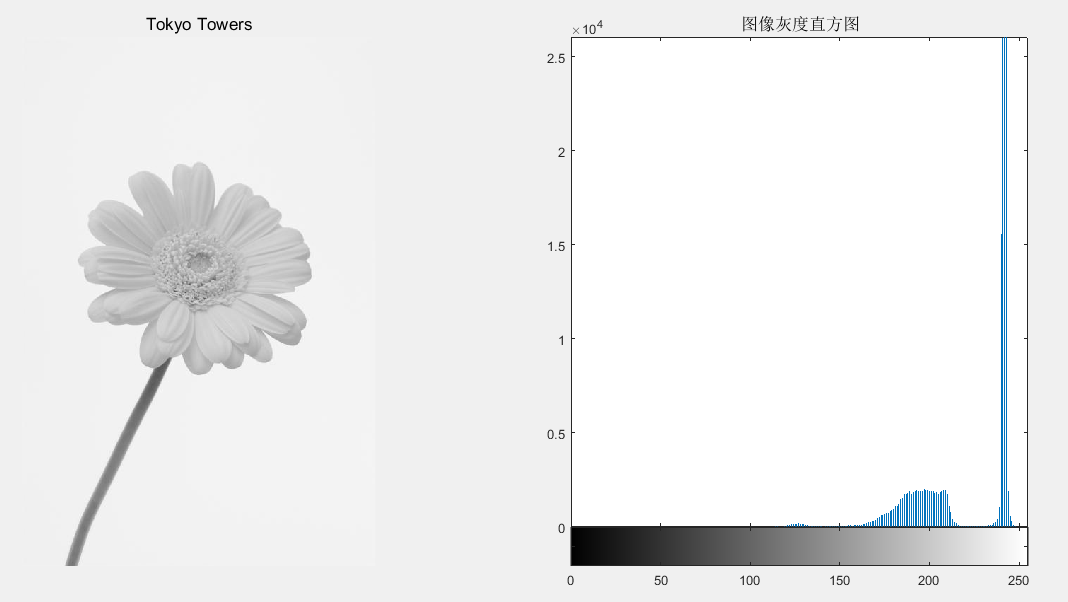## 5、图像分割（边缘提取）

### 1）图像几何纠正、边缘检测

B=imresize(A,m,type): 图像大小调整

B=imrotate(A,angle,method): 图像旋转调整

I=rgb2gray(InImg);
B1=imresize(I,1.5, 'nearest');
B2=imresize(I,1.5, 'bilinear');
C1= imrotate (I,45, 'nearest');
C2= imrotate (I,45, 'bilinear');
subplot(231);imshow(I) ;%显示原始图像于
title('原始图像');
subplot(232); imshow(B1) ;%显示调整图像(最近邻插值)
title('最近邻插值图像');
subplot(233); imshow(B2) ;% 显示调整图像(双线性插值)
title('双线性插值图像');
subplot(234); imshow(C1);% 显示旋转图像(最近邻插值)
title('旋转最近邻插值图像');
subplot(235); imshow(C2);% 显示旋转图像(双线性插值)
title('旋转双线性插值');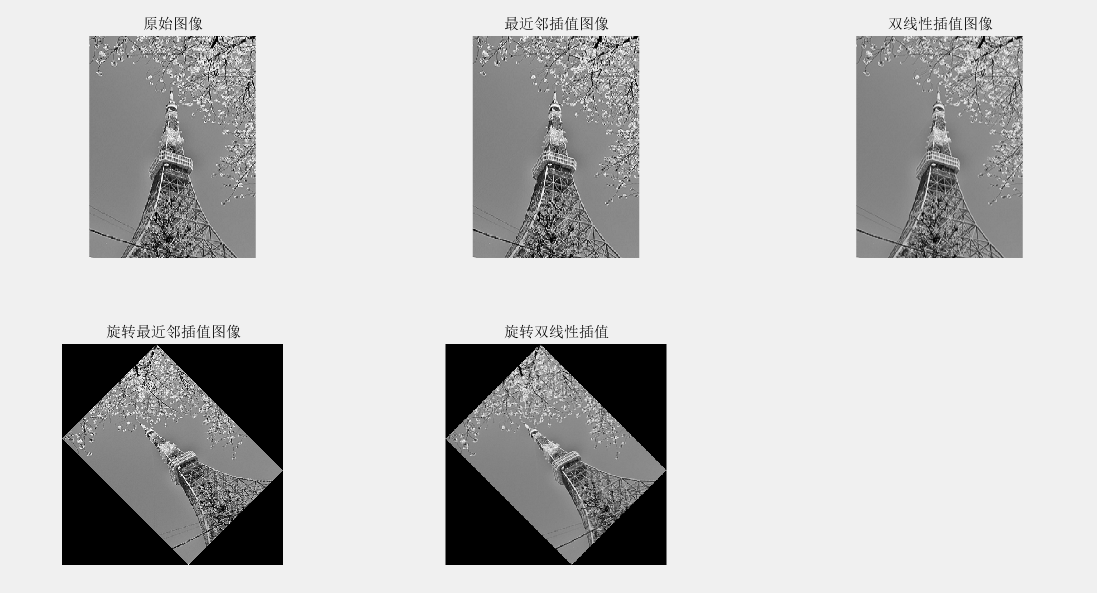2）边缘提取

BW=edge(I,method,thresh): 检测灰度图像的边缘

I=rgb2gray(InImg);
BW1= edge (I, 'sobel',0.1);%sobel边缘检测器
BW2= edge (I, 'prewitt',0.1);%prewitt边缘检测器
BW3= edge (I, 'roberts',0.1);%roberts边缘检测器
BW4= edge (I, 'log',0.01,2.0);%log边缘检测器
subplot(221); imshow(BW1) ;% 显示边缘图像
title('sobel边缘图像');
subplot(222); imshow(BW2) ;%显示边缘图像
title('prewitt边缘图像');
subplot(223); imshow(BW3) ;% 显示边缘图像
title('roberts边缘图像');
subplot(224); imshow(BW4) ;% 显示边缘图像
title('log边缘图像');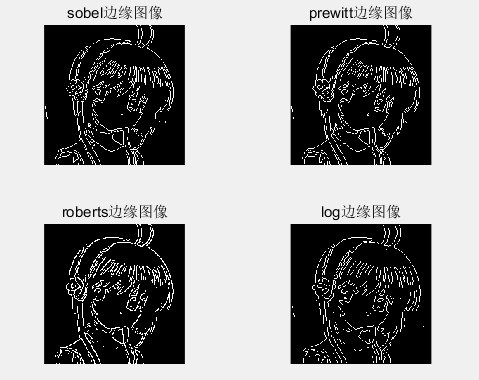## 边缘提取

2016-10-08 21:57:43 jiandanokok 阅读数 19943
• ###### MATLAB图像处理

全面系统的学习MATLAB在图像处理中的应用

19908 人正在学习 去看看 魏伟

# 一、引入图像模块

1. PIL简介：PIL(Python Imaging Library Python,图像处理类库)提供了通用的图像处理功能,以及大量有用的基本图像操作,比如图像缩放、裁剪、旋转、颜色转换等。利用 PIL 中的函数,我们可以从大多数图像格式的文件中读取数据,然后写入最常见的图像格式文件中。PIL 中最重要的模块为 Image

2. 引入PIL：
from PIL import Image

# 二、读取与存储操作

1. PIL 的 open() 函数用于创建 PIL 图像对象，比如读取一张图片：
# coding=utf-8from PIL import Image
# 读取图片img = Image.open('qq_image.jpg')

2. 存储图片：
save() 方法用于保存图像到具有指定文件名的文件。通过 save() 方法，PIL 可以将图像保存成多种格式的文件，PIL 是个足够智能的类库，可以根据文件扩展名来判定图像的格式。比如：img.save('qq_image_thumb.jpg', 'JPEG')，PIL 函数会进行简单的检查，如果文件不是 JPEG 格式，会自动将其转换成 JPEG 格式；如果转换失败，它会在控制台输出一条报告失败的消息。

# coding=utf-8from PIL import Image
# 读取图片img = Image.open('qq_image.jpg')
# 生成缩略图img.thumbnail((128, 128))
# 保存图片img.save('qq_image_thumb.jpg', 'JPEG')# 三、图像的一些基本操作

### 1. 输出图片的信息（格式、尺寸以及图像类型）

# coding=utf-8from PIL import Image
# 读取图片img = Image.open('qq_image.jpg')
# 输出图片的格式，尺寸以及图像类型print img.format, img.size, img.mode

### 2. 显示图片

# coding=utf-8from PIL import Image
# 读取图片img = Image.open('qq_image.jpg')
# 显示图片img.show()
PyCharm下运行呢，会直接调用Windows照片查看器来显示图片

### 3. 生成缩略图

thumbnail() 方法接受一个元组参数（该参数指定生成缩略图的大小），然后将图像转换成符合元组参数指定大小的缩略图。
# coding=utf-8from PIL import Image
# 读取图片img = Image.open('qq_image.jpg')
# 生成缩略图img.thumbnail((128, 128))
# 显示图片img.show()

### 4. 旋转图片

# coding=utf-8from PIL import Image
# 读取图片img = Image.open('qq_image.jpg')
# 逆时针旋转45度rorate1 = img.rotate(45)rorate1.show()
# 逆时针旋转90rorate2 = img.transpose(Image.ROTATE_90)rorate2.show()

rorate1：rorate2：### 5. 翻转

# coding=utf-8from PIL import Image
# 读取图片img = Image.open('qq_image.jpg')
# 左右对换rorate3 = img.transpose(Image.FLIP_LEFT_RIGHT)rorate3.show()
# 上下翻转rorate4 = img.transpose(Image.FLIP_TOP_BOTTOM)rorate4.show()

rorate3:rorate4:### 6. 缩放图片

# coding=utf-8from PIL import Image
# 读取图片img = Image.open('qq_image.jpg')
# 缩放图片为50*50resize1 = img.resize((50, 50))resize1.show()

### 7. 图片灰度化

# coding=utf-8from PIL import Image
# 读取图片img = Image.open('qq_image.jpg')
# 图片转换为灰度grey = img.convert('L')grey.show()### 8. 某个像素点的色彩值的获取以及更改

# coding=utf-8from PIL import Image
# 读取图片img = Image.open('qq_image.jpg')
# 获取某个像素位置的值print img.getpixel((100, 100))
# 更改某个像素位置的值img.putpixel((100, 100), (0, 0, 0))

### 9. 裁剪图片

# coding=utf-8from PIL import Image
# 读取图片img = Image.open('qq_image.jpg')
# 裁剪图片  box为裁剪图片的区域范围box = (100, 100, 250, 250)region = img.crop(box)region.show()注意：Python规定左上角为(0, 0)的坐标点，box由一个4元组(左，上，右，下)定义，表示为坐标为： (left, upper, right, lower)，最后的两个数字必须比前面两个要大。如图：### 10. 粘贴图片

# coding=utf-8from PIL import Image
# 读取图片img = Image.open('qq_image.jpg')
# 裁剪图片  box为裁剪图片的区域范围box = (100, 100, 250, 250)region = img.crop(box)region.show()
# 逆时针旋转图片180度region2 = region.transpose(Image.ROTATE_180)region2.show()
# 图片的粘贴img.paste(region2, box)img.show()## 总结：

2019-06-30 14:14:03 great_name 阅读数 259
• ###### MATLAB图像处理

全面系统的学习MATLAB在图像处理中的应用

19908 人正在学习 去看看 魏伟

# 第一章

1.图像获取； 2.图像增强； 3.图像修复； 4.形态学处理； 5.分割； 6.对象识别； 7.描述； 8.图像压缩； 9.彩色图像处理

# 第二章

BIP:以像元为基本单位（pixel）；BIL按照扫描行为的单位（line）；BSQ：以波段为单位；BMP由文件头、位图信息、位图数据三部分组成；

ps：采样间隔与空间分辨率有关，量化级数与辐射分辨率有关，因为能量守恒，空间分辨率越高，每个传感器收到的能量就越小，量化级数就很难提高。

# 第三章

p.s几何多项式模型不适合用来校正地形起伏较大的遥感影像，因为几何多项式模型的输入为x、y坐标，缺少高程信息，所以对高程的校正效果很差

# 第五章

p.s sobel梯度是在prewitt算法的基础上，对4邻域采用加权方法进行差分，因而对边缘的检测更加精确。

一：理解空间信息

# 第八章

• 基于边缘检测的分割：先提取边界区域，再确定边界限定的区域；
• 区域分割：确定每一个像素的归属区域，从而形成一个区域图；
• 区域生长：将属性相近的连通像素聚集成区域；
• 分裂——合并分割：综合利用前两种方法，即存在图像的划分，又存在图像的合并；

OSTU方法的原理：OSTU（大津法）寻找一个阈值，使类间方差最大化，通过这个阈值来分割。大津法的关键是找到最大类间方差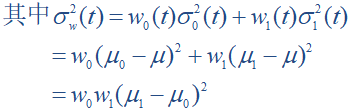2016-06-06 17:34:31 xueyedie1234 阅读数 23289
• ###### MATLAB图像处理

全面系统的学习MATLAB在图像处理中的应用

19908 人正在学习 去看看 魏伟

### 文章目录

# 一、 什么是卷积？       在图像处理中，卷积操作指的是使用一个卷积核对图像中的每个像素进行一系列操作。       卷积核（算子）是用来做图像处理时的矩阵,图像处理时也称为掩膜，是与原图像做运算的参数。卷积核通常是一个四方形的网格结构（例如3*3的矩阵或像素区域），该区域上每个方格都有一个权重值。       使用卷积进行计算时，需要将卷积核的中心放置在要计算的像素上，一次计算核中每个元素和其覆盖的图像像素值的乘积并求和，得到的结构就是该位置的新像素值。       以下两个算子中演示了具体的卷积计算过程。
# 二、 相关算子 定义：![这里写图片描述](http://img.blog.csdn.net/20160603125126517) 即![这里写图片描述](http://img.blog.csdn.net/20160603125155767)，其中ｈ称为相关核(Kernel). 　　　　　　　　 步骤：
• 1）滑动核，使其中心位于输入图像g的（i，j）像素上
• 2）利用上式求和，得到输出图像的（i，j）像素值
• 3）充分上面操纵，直到求出输出图像的所有像素值

【例】
原始像素矩阵为：
\begin{bmatrix}
17 & 24 & 1 & 8 & 15 \
23 & 5 & 7 & 14 & 16 \
4 & 6 & 13 & 20 & 22 \
10 & 12 & 19 & 21 & 3\
11 & 18 & 25 & 2 & 9
\end{bmatrix}

\begin{bmatrix}
8 & 1 & 6 \
3 & 5 & 7 \
4 & 9 & 2
\end{bmatrix}# 三、 卷积算子• 1）将核围绕中心旋转180度
• 2）滑动核，使其中心位于输入图像g的（i，j）像素上
• 3）利用上式求和，得到输出图像的（i，j）像素值
• 4）充分上面操纵，直到求出输出图像的所有像素值
例：计算输出图像的（2，4）元素=12+89+154+77+145+163+136+201+22*8=575
如图所示：# 四、 边缘效应       当对图像边缘的进行滤波时，核的一部分会位于图像边缘外面。 ![这里写图片描述](http://img.blog.csdn.net/20160603143253118) 常用的策略包括： - 1）使用常数填充：imfilter默认用0填充，这会造成处理后的图像边缘是黑色的。 - 2）复制边缘像素：I3 = imfilter(I,h,'replicate'); ![这里写图片描述](http://img.blog.csdn.net/20160603143353525)
# 五、 常用的卷积核及其用途
• 1）低通滤波器（常用于计算模糊后的效果）\begin{bmatrix}
1/9 & 1/9 & 1/9 \
1/9 & 1/9 & 1/9 \
1/9 & 1/9 & 1/9
\end{bmatrix}
\begin{bmatrix}
1/10 & 1/10 & 1/10 \
1/10 & 2/10 & 1/10 \
1/10 & 1/10 & 1/10
\end{bmatrix}
\begin{bmatrix}
1/16 & 2/16 & 1/16 \
2/16 & 4/16 & 2/16 \
1/16 & 2/16 & 1/16
\end{bmatrix}

• 2）高斯滤波器（常用于计算高斯模糊后的效果）
高斯模糊的卷积核也是一个正方形的滤波核，其中每个元素通过以下公式计算得出：
$G(x,y)=\frac{1}{2πσ^{2}}·e^{\frac{x^{2}+y^{2}}{2σ^{2}}}$
该公式中σ是标准方差（一般取值为1），x和y分别对应了当前位置到卷积核中心的整数距离。通过这个公式，就可以计算出高斯核中每个位置对应的值。为了保证滤波后的图像不会变暗，需要对高斯核中的权重进行归一化。

• 3）边缘检测（常用于计算图像边缘或者说梯度值）
\begin{bmatrix}
-1 & 0 & -1 \
0 & 4 & 0 \
-1 & 0 & -1
\end{bmatrix}

# 六、 一个例子——使用卷积实现模糊效果       我们将对下面这张图进行模糊处理： ![这里写图片描述](http://img.blog.csdn.net/20160606172529227)       以下为compute shader中关于卷积处理的代码：
[numthreads(32,32,1)]
void Dim_Main (uint3 id : SV_DispatchThreadID)
{

float sumR = 0;
float sumG = 0;
float sumB = 0;
float sumA = 0;
for (int i = -1; i <= 1; i++)
{
for (int j = -1; j <= 1; j++)
{
sumR += texBuffer[(id.x+i)*texWidth+(id.y+j)].r * convolutionTempBuffer[(i+1)*3+(j+1)];
sumG += texBuffer[(id.x+i)*texWidth+(id.y+j)].g * convolutionTempBuffer[(i+1)*3+(j+1)];
sumB += texBuffer[(id.x+i)*texWidth+(id.y+j)].b * convolutionTempBuffer[(i+1)*3+(j+1)];
sumA += texBuffer[(id.x+i)*texWidth+(id.y+j)].a * convolutionTempBuffer[(i+1)*3+(j+1)];
}
}

texBuffer[id.x*texWidth+id.y].r = sumR;
texBuffer[id.x*texWidth+id.y].g = sumG;
texBuffer[id.x*texWidth+id.y].b = sumB;
texBuffer[id.x*texWidth+id.y].a = sumA;

Result[id.xy] = float4(sumR, sumG, sumB, sumA);
}图中可以明显的看到左右两边有明显的黑色线条，原图中是没有这样的黑色的，产生这种效果的原因是本文中之前提到过的边缘效应。下面我将修改一部分代码去除边缘效应带来的影响，这里使用的是相邻像素的值方法。

[numthreads(32,32,1)]
void Dim_Main (uint3 id : SV_DispatchThreadID)
{

float sumR = 0;
float sumG = 0;
float sumB = 0;
float sumA = 0;
for (int i = -1; i <= 1; i++)
{
for (int j = -1; j <= 1; j++)
{
if((id.x+i)*texWidth+(id.y+j)>texWidth*texWidth-1)
{
sumR += texBuffer[(id.x+i)*texWidth+(id.y+j)-texWidth].r * convolutionTempBuffer[(i+1)*3+(j+1)];
sumG += texBuffer[(id.x+i)*texWidth+(id.y+j)-texWidth].g * convolutionTempBuffer[(i+1)*3+(j+1)];
sumB += texBuffer[(id.x+i)*texWidth+(id.y+j)-texWidth].b * convolutionTempBuffer[(i+1)*3+(j+1)];
sumA += texBuffer[(id.x+i)*texWidth+(id.y+j)-texWidth].a * convolutionTempBuffer[(i+1)*3+(j+1)];
}

sumR += texBuffer[(id.x+i)*texWidth+(id.y+j)].r * convolutionTempBuffer[(i+1)*3+(j+1)];
sumG += texBuffer[(id.x+i)*texWidth+(id.y+j)].g * convolutionTempBuffer[(i+1)*3+(j+1)];
sumB += texBuffer[(id.x+i)*texWidth+(id.y+j)].b * convolutionTempBuffer[(i+1)*3+(j+1)];
sumA += texBuffer[(id.x+i)*texWidth+(id.y+j)].a * convolutionTempBuffer[(i+1)*3+(j+1)];
}
}

texBuffer[id.x*texWidth+id.y].r = sumR;
texBuffer[id.x*texWidth+id.y].g = sumG;
texBuffer[id.x*texWidth+id.y].b = sumB;
texBuffer[id.x*texWidth+id.y].a = sumA;

Result[id.xy] = float4(sumR, sumG, sumB, sumA);
}可以看到，图中左边的黑色线条已经被滤除，右边也可以采用类似的方法来剔除。实际使用中，也可以根据情况使用纯色来做剔除，这样可以节省部分效率，如下图中我使用的是纯白色来剔除边缘效应。2010-07-23 17:10:00 Jamie406 阅读数 921
• ###### MATLAB图像处理

全面系统的学习MATLAB在图像处理中的应用

19908 人正在学习 去看看 魏伟

最近在学习C语言图像处理，真的很郁闷，感觉摸不到门道，而且老师要求尽快掌握，还要处理一些图像数据，只能在网上找找现成的消化下，希望能有助有学习和理解。

C语言图像处理方法
1．图像平移
图像平移只是改变图像在屏幕上的位置，图像本身并不发生变化。

1．图像平移
图像平移只是改变图像在屏幕上的位置，图像本身并不发生变化。
假设原图像区域左上角坐标为(x0, y0)，右下角坐标为(x1, y1)，将图像分别沿x和y轴平移dx和dy，

x′ = x ＋ dx
y′ = y ＋ dy
在屏幕上实现图像的移动分为四个步骤：
⑴ 保存原图像到缓冲区。
⑵ 擦除原图像。
⑶ 计算平移后的新坐标。
⑷ 在新的坐标位置重新显示原图像。
其中，擦除原图像的方法与图形变换中擦除原图形的方法一致，在实现中仍采用XOR异或方式画图擦

2．图像颠倒
图像颠倒是指把定义好的图像区域上下翻转地显示在屏幕上。分析图像颠倒的过程，可发现每行的图

(1) 用getimage()保存原图像，并擦除原图像。
(2) 计算图像的高度，即行数height；计算图像宽度width；计算保存一行图像信息
height = bottom － top ＋ 1;
width = right － left ＋ 1;
linebytes = (width ＋ 7) ／ 8 ＊ 4;
(3)利用行交换缓冲区linebuf在图像内存缓冲区中进行信息交换，即把第一行与最末行交换，第2行与

(4)把交换后的图像缓冲区内容重新显示在屏幕上。
3．图像镜像变换
镜像变换是指将指定区域的图像左右翻转地显示在屏幕。分析镜像变换过程可以发现：每行图像信息

首先，对于左上角为（left, top)，右下角为（right, bottom)矩形区域图像，给出其中任意点（x0, y0）

x′ = right － x0 ＋ left
y′ = y0
根据以上公式，对各个像素点计算新坐标后，直接把它显示在屏幕的相应位置上。
如果完全逐点地进行交换，处理一个像素点就要读取一次像素值，从而降低了变换速度。由于像素点是顺

将一矩形区域的图像进行镜像变换的基本步骤如下：
(1) 用getimage()把图像保存到内存缓冲区，并擦除原图像。
(2) 计算图像高度，即行数高度height和宽度width；计算保存一行图像信息占用的字节数linebytes。计

height = bottom － top ＋ 1;
width = right － left ＋ 1;
linebyte = (width ＋ 7) ／ 8 ＊4;
(3) 对图像进行镜像。
(4)释放内存图像缓冲区。
4．图像旋转
图像旋转是指把定义的图像绕某一点以逆时针或顺时针方向旋转一定的角度，通常是指绕图像的中心以逆

假设图像的左上角为（left, top),右下角为（right, bottom)，则图像上任意点（x0, y0）绕其中心
（xcenter, ycenter)逆时针旋转angle角度后，新的坐标位置（x′, y′）的计算公式为：
xcenter = (right － left ＋ 1) ／ 2 ＋ left;
ycenter = (bottom － top ＋ 1) ／ 2 ＋ top;
x′ = (x0 － xcenter) cosθ － (y0 － ycenter) sinθ ＋ xcenter;
y′ = (x0 － xcenter) sinθ ＋ (y0 － ycenter) cosθ ＋ ycenter;
与图像的镜像变换相类似，也采用按行逐点变换的方式实现图像的旋转，其步骤如下：
(1) 用getimage（）把图像保存到内存缓冲区，并擦除原图像。
(2) 计算图像高度height，宽度width，及保存一行图像信息占用的字节数linebytes，计算公式与镜像变

(3) 对图像逐行进行旋转变换。
(4) 释放内存图像缓冲区。
值得指出的是，这种处理方法不够。为此可以采用另一种方法：先在图像变换缓冲区中处理完毕后，再将